# Contest Winner Draw Method (EuroMillions)

 How it works Example A number will be assigned to each entry in the order posted beginning with the number zero. (Each entrant’s name and Designated Number (DN) will be added/updated in the first post in the event thread) Post = 0 Post = 1 Post = 2 ... 25. Post = 25 Every Friday the EuroMillions Lottery generates 5 numbers + 2 lucky star numbers that are published at EuroMillions Results. These 7 numbers drawn on the first Friday after the deadline of the contest will be used to determine the winner.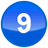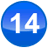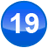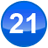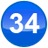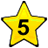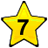An integer is formed from the drawn numbers and lucky star numbers. 9, 14, 19, 21, 34, 5 and 7 would give 91419213457. Then the Modulo operation will be used to calculate the winning number as follows: Mod = The Modulo operation results in the "Modulo" - the remainder on a division of one number by another number. This modulo represent the designated number of the winning entry. 91419213457 Mod 25 = 7 The Designated Number of the winning entry is 7. An easy way to calculate the "Modulo" is the Windows Calculator: Start -> Programs -> Accessories -> Calculator open the calculator enter "91419213457" click on the "Mod" key enter "25" press the equal symbol (or "Enter" on your keyboard) read the result: "7" If more than one winner is to be determined (1st, 2nd, 3rd place) then the divisor in the Modulo operation is dropped by 1, and then 2, and so forth to determine all winners: Mod = Mod = Mod = ... 91419213457 Mod 25 = 7 (= 1st place) 91419213457 Mod 24 = 1 (= 2nd place) 91419213457 Mod 23 = 4 (= 3rd place) ...